Examples

Chapter 3 Class 10 Pair of Linear Equations in Two Variables (Term 1)
Serial order wise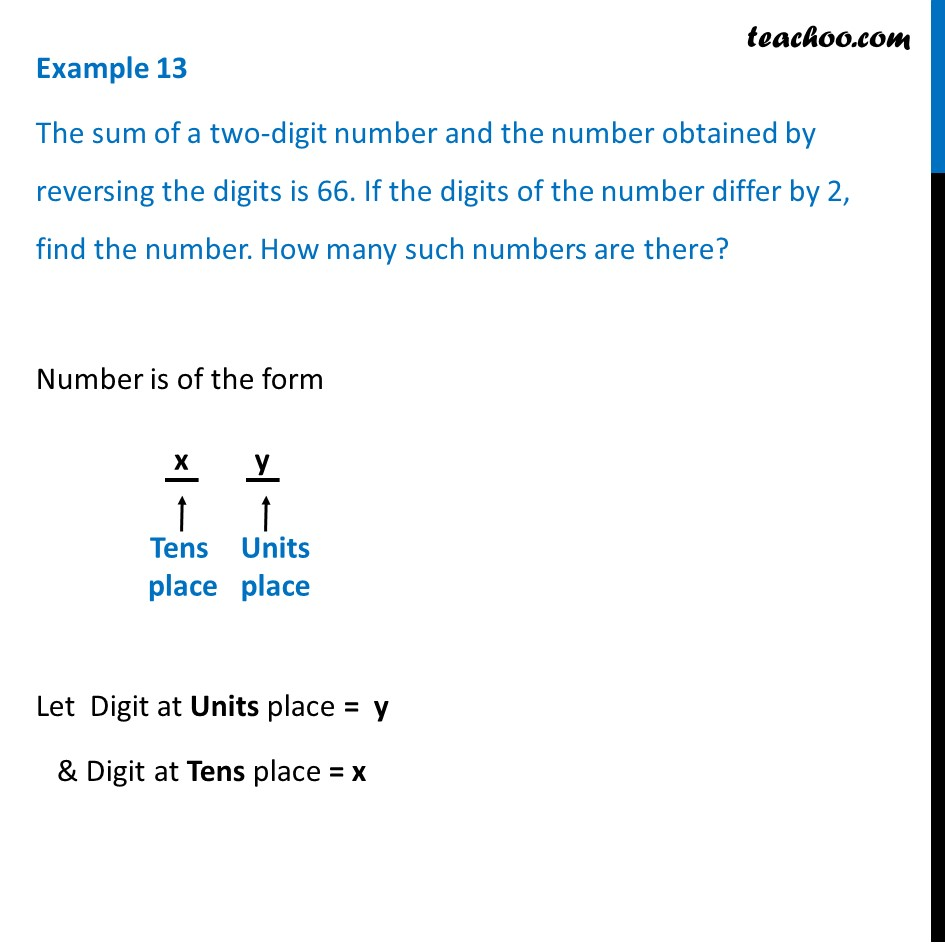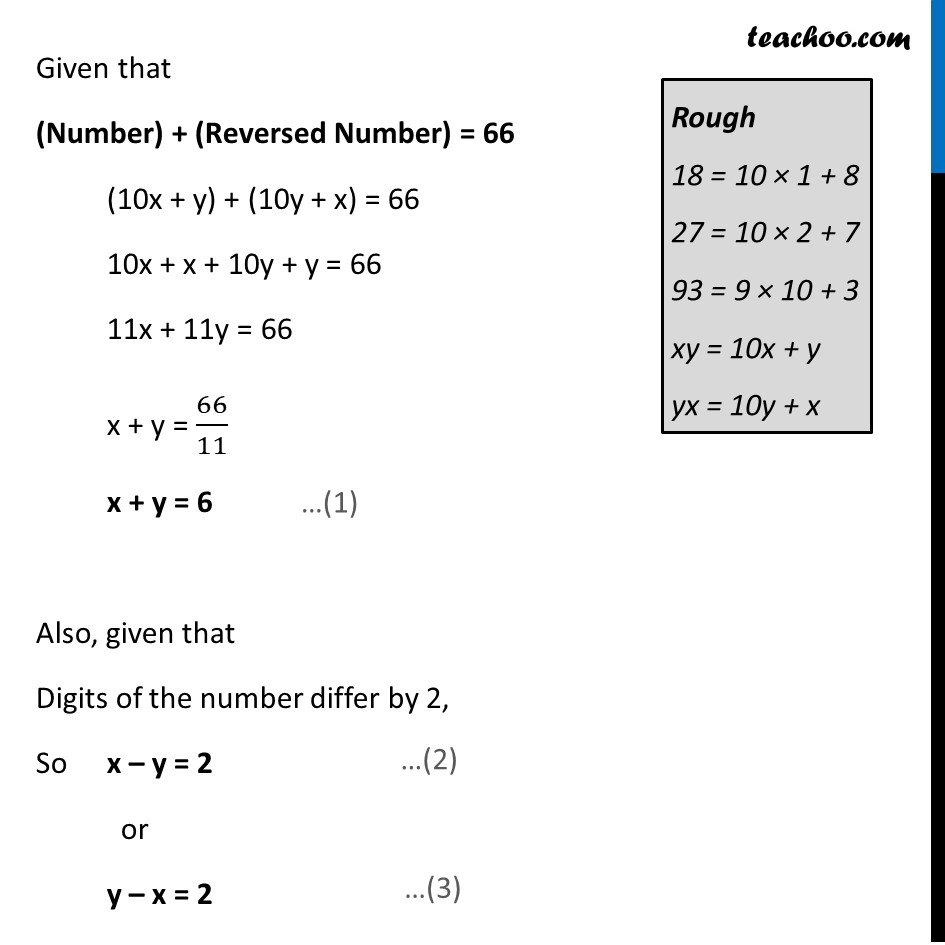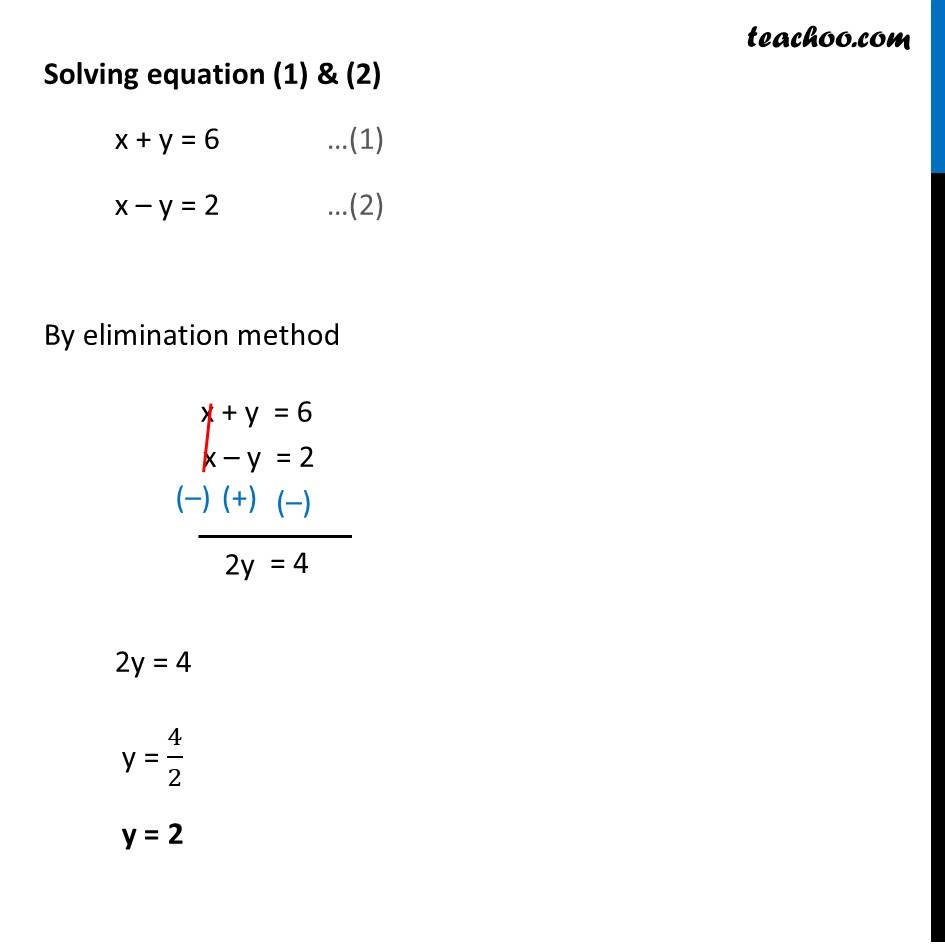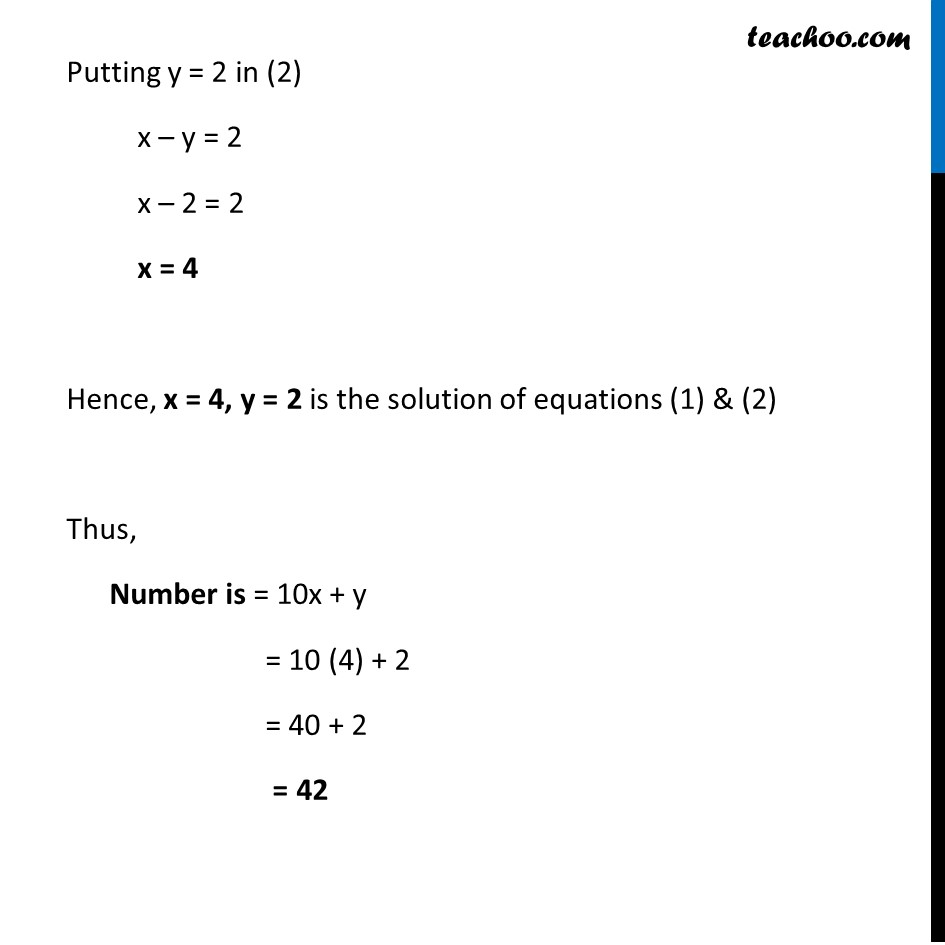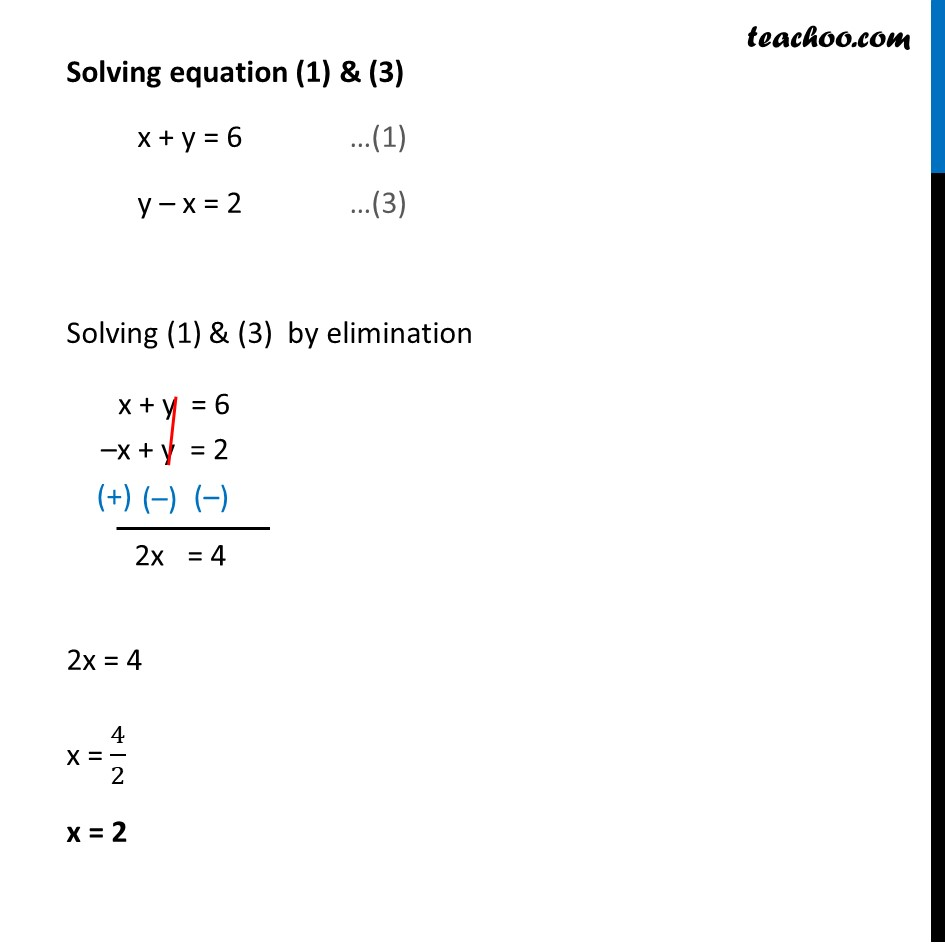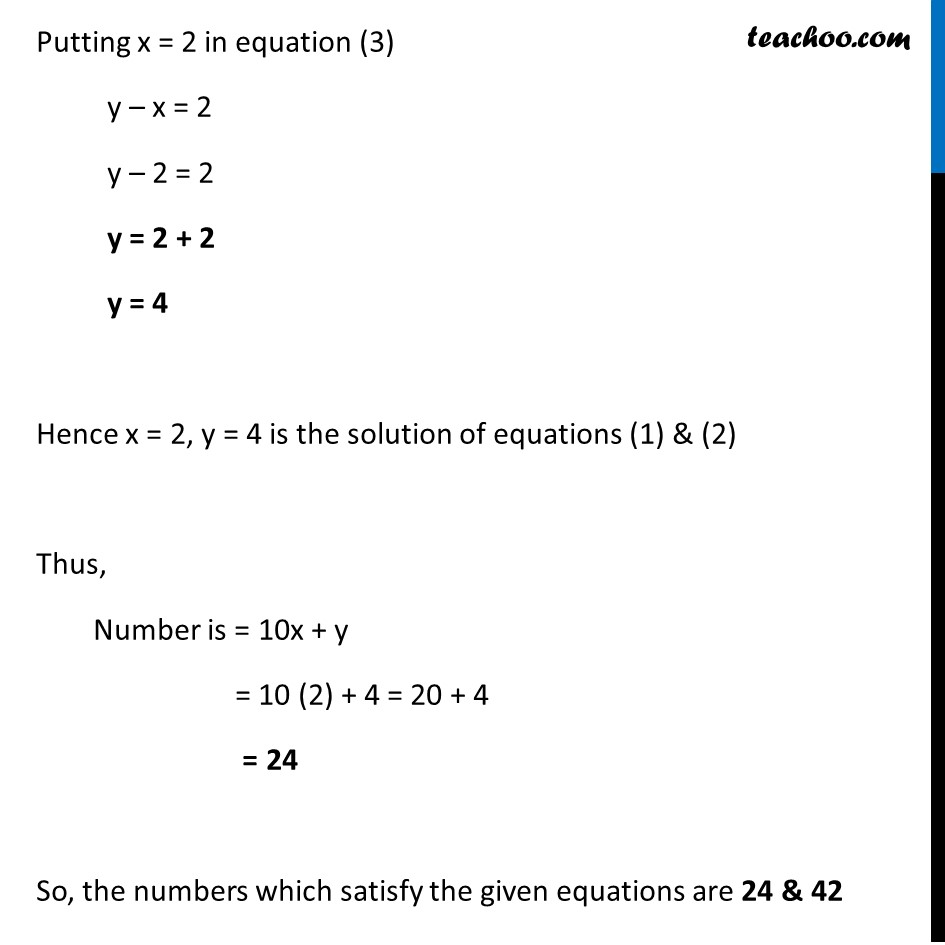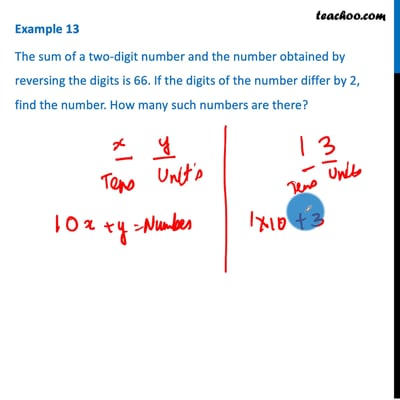This video is only available for Teachoo black users

### Transcript

Example 13 The sum of a two-digit number and the number obtained by reversing the digits is 66. If the digits of the number differ by 2, find the number. How many such numbers are there? Number is of the form Let Digit at Units place = y & Digit at Tens place = x Given that (Number) + (Reversed Number) = 66 (10x + y) + (10y + x) = 66 10x + x + 10y + y = 66 11x + 11y = 66 x + y = 66/11 x + y = 6 Also, given that Digits of the number differ by 2, So x – y = 2 or y – x = 2 Solving equation (1) & (2) x + y = 6 …(1) x – y = 2 …(2) By elimination method 2y = 4 y = 4/2 y = 2 Putting y = 2 in (2) x – y = 2 x – 2 = 2 x = 4 Hence, x = 4, y = 2 is the solution of equations (1) & (2) Thus, Number is = 10x + y = 10 (4) + 2 = 40 + 2 = 42 Solving equation (1) & (3) x + y = 6 …(1) y – x = 2 …(3) Solving (1) & (3) by elimination 2x = 4 x = 4/2 x = 2 Putting x = 2 in equation (3) y – x = 2 y – 2 = 2 y = 2 + 2 y = 4 Hence x = 2, y = 4 is the solution of equations (1) & (2) Thus, Number is = 10x + y = 10 (2) + 4 = 20 + 4 = 24 So, the numbers which satisfy the given equations are 24 & 42